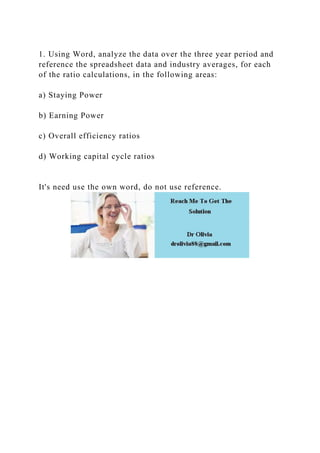Successfully reported this slideshow.

# 1. Using Word, analyze the data over the three year period and refer.docx×

# 1. Using Word, analyze the data over the three year period and refer.docx

1. Using Word, analyze the data over the three year period and reference the spreadsheet data and industry averages, for each of the ratio calculations, in the following areas:

a) Staying Power

b) Earning Power

c) Overall efficiency ratios

d) Working capital cycle ratios

It's need use the own word, do not use reference.
.

1. Using Word, analyze the data over the three year period and reference the spreadsheet data and industry averages, for each of the ratio calculations, in the following areas:

a) Staying Power

b) Earning Power

c) Overall efficiency ratios

d) Working capital cycle ratios

It's need use the own word, do not use reference.
.